Anúncio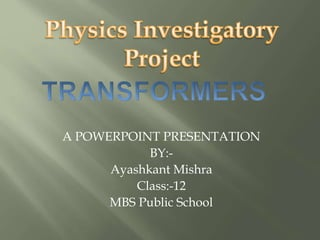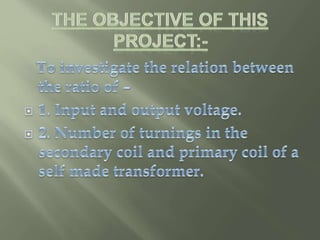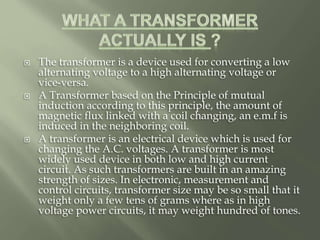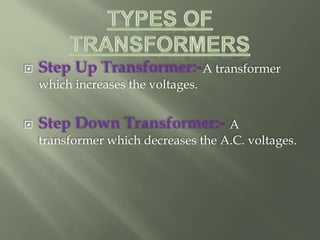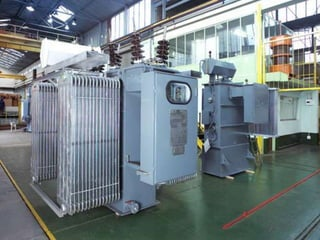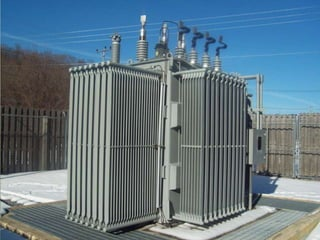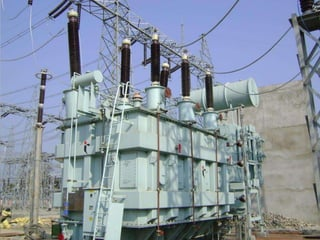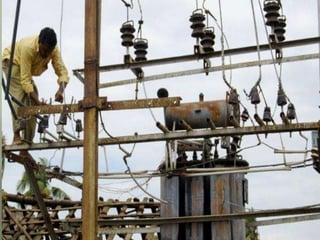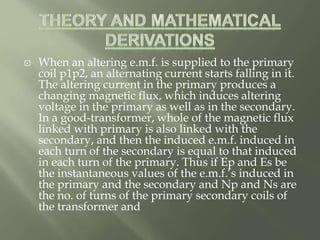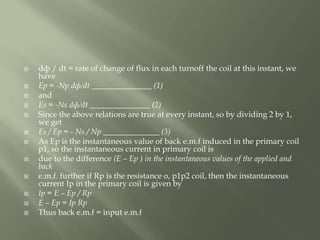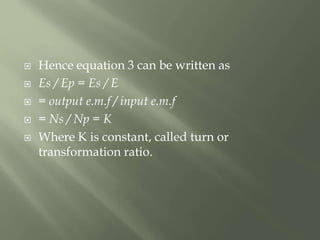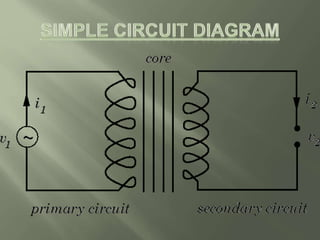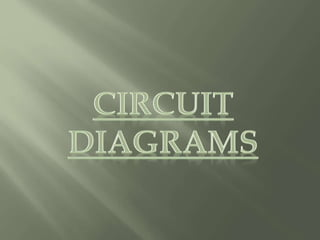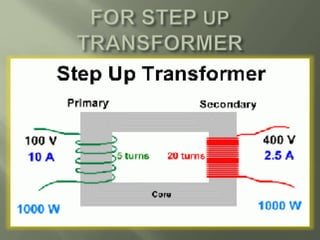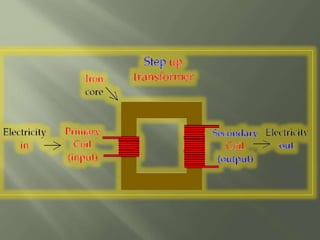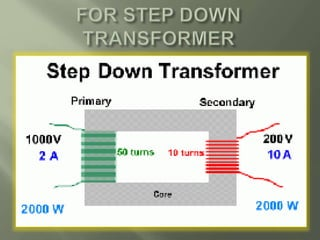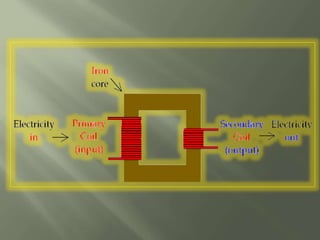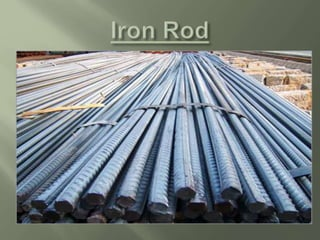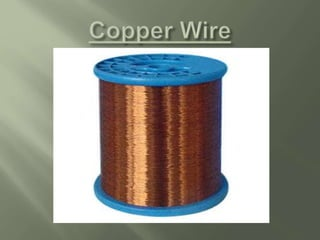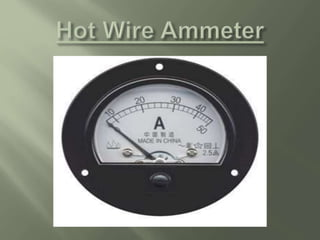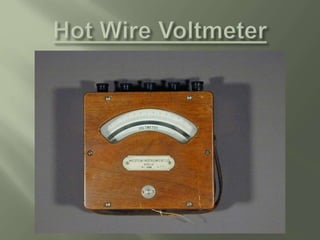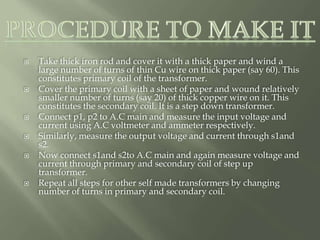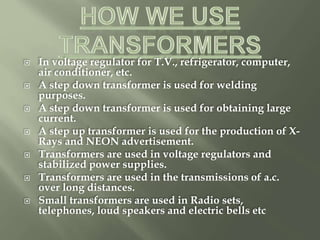1 de 27
Anúncio

### transformers ppt class 12

1. A POWERPOINT PRESENTATION BY:- Ayashkant Mishra Class:-12 MBS Public School
2.  The transformer is a device used for converting a low alternating voltage to a high alternating voltage or vice-versa.  A Transformer based on the Principle of mutual induction according to this principle, the amount of magnetic flux linked with a coil changing, an e.m.f is induced in the neighboring coil.  A transformer is an electrical device which is used for changing the A.C. voltages. A transformer is most widely used device in both low and high current circuit. As such transformers are built in an amazing strength of sizes. In electronic, measurement and control circuits, transformer size may be so small that it weight only a few tens of grams where as in high voltage power circuits, it may weight hundred of tones.
3.  Step Up Transformer:-A transformer which increases the voltages.  Step Down Transformer:- A transformer which decreases the A.C. voltages.
4.  When an altering e.m.f. is supplied to the primary coil p1p2, an alternating current starts falling in it. The altering current in the primary produces a changing magnetic flux, which induces altering voltage in the primary as well as in the secondary. In a good-transformer, whole of the magnetic flux linked with primary is also linked with the secondary, and then the induced e.m.f. induced in each turn of the secondary is equal to that induced in each turn of the primary. Thus if Ep and Es be the instantaneous values of the e.m.f.’s induced in the primary and the secondary and Np and Ns are the no. of turns of the primary secondary coils of the transformer and
5.  dф / dt = rate of change of flux in each turnoff the coil at this instant, we have  Ep = -Np dф/dt _______________ (1)  and  Es = -Ns dф/dt _______________ (2)  Since the above relations are true at every instant, so by dividing 2 by 1, we get  Es / Ep = - Ns / Np ______________ (3)  As Ep is the instantaneous value of back e.m.f induced in the primary coil p1, so the instantaneous current in primary coil is  due to the difference (E – Ep ) in the instantaneous values of the applied and back  e.m.f. further if Rp is the resistance o, p1p2 coil, then the instantaneous current Ip in the primary coil is given by  Ip = E – Ep / Rp  E – Ep = Ip Rp  Thus back e.m.f = input e.m.f
6.  Hence equation 3 can be written as  Es / Ep = Es / E  = output e.m.f / input e.m.f  = Ns / Np = K  Where K is constant, called turn or transformation ratio.
7.  Take thick iron rod and cover it with a thick paper and wind a large number of turns of thin Cu wire on thick paper (say 60). This constitutes primary coil of the transformer.  Cover the primary coil with a sheet of paper and wound relatively smaller number of turns (say 20) of thick copper wire on it. This constitutes the secondary coil. It is a step down transformer.  Connect p1, p2 to A.C main and measure the input voltage and current using A.C voltmeter and ammeter respectively.  Similarly, measure the output voltage and current through s1and s2.  Now connect s1and s2to A.C main and again measure voltage and current through primary and secondary coil of step up transformer.  Repeat all steps for other self made transformers by changing number of turns in primary and secondary coil.
8.  In voltage regulator for T.V., refrigerator, computer, air conditioner, etc.  A step down transformer is used for welding purposes.  A step down transformer is used for obtaining large current.  A step up transformer is used for the production of X- Rays and NEON advertisement.  Transformers are used in voltage regulators and stabilized power supplies.  Transformers are used in the transmissions of a.c. over long distances.  Small transformers are used in Radio sets, telephones, loud speakers and electric bells etc
Anúncio